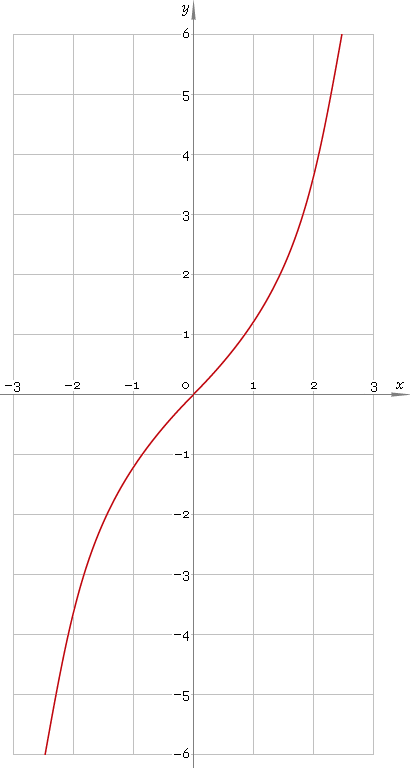The Art of Interface

# sinh or sh — hyperbolic sine function

Category. Mathematics.

Abstract. Hyperbolic sine: definition, plot, properties and identities.

## 1. Definition

Hyperbolic sine is defined as

sinhx ≡ (ex − ex) /2

## 2. Plot

Hyperbolic sine is antisymmetric function defined everywhere on real axis. Its plot is depicted below — fig. 1.Fig. 1. Plot of the hyperbolic sine function y = sinhx.

Function codomain is entire real axis.

## 3. Identities

Base:

cosh2x − sinh2x = 1

Connection to exponential function:

sinhx + coshx = ex
coshx − sinhx = ex

By definition:

sinhx ≡ 1 /cschx

Property of antisymmetry:

sinh−x = −sinhx

Half-argument:

sinh(x/2) = √[(coshx − 1) /2]
sinhx = 2 tanh(x/2) /[1 − tanh2(x/2)]

Double argument:

sinh(2x) = 2 sinhx coshx

Triple argument:

sinh(3x) = 4 sinh3x + 3 sinhx

sinh(4x) = 4 sinh3x coshx + 4 sinhx cosh3x

Power reduction:

sinh2x = (cosh(2x) − 1) /2
sinh3x = (sinh(3x) − 3 sinhx) /4
sinh4x = (cosh(4x) − 4 cosh(2x) + 3) /8
sinh5x = (sinh(5x) − 5 sinh(3x) + 10 sinhx) /16

Sum and difference of arguments:

sinh(x + y) = sinhx coshy + coshx sinhy
sinh(xy) = sinhx coshy − coshx sinhy

Product-to-sum:

sinhx sinhy = [cosh(x + y) − cosh(xy)] /2
sinhx coshy = [sinh(x + y) + sinh(xy)] /2

Sum-to-product:

sinhx + sinhy = 2 sinh[(x + y) /2] cosh[(xy) /2]
sinhx − sinhy = 2 sinh[(xy) /2] cosh[(x + y) /2]

## 4. Support

Hyperbolic sine function sinh or sh of the real argument is supported by free version of the Librow calculator.

Hyperbolic sine function sinh or sh of the complex argument is supported by professional version of the Librow calculator.

## 5. How to use

To calculate hyperbolic sine of the number:

``sinh(-1);``

To calculate hyperbolic sine of the current result:

``sinh(rslt);``

To calculate hyperbolic sine of the number x in memory:

``sinh(mem[x]);``